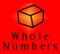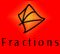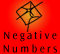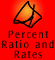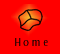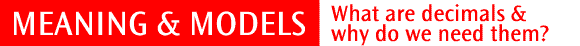| The part-whole relationship | Recording and naming decimals | Basic Equivalences |
| Models of decimals | Quick Quiz |

The part-whole relationship

Like common fractions, decimals enable us to describe parts of a complete unit. Fractions and decimals are different ways of writing numbers. Both systems have advantages. With fractions, the numerator and denominator show directly the number of parts and the size of the parts. Decimals, however, show only the number of parts, and we determine the size of those parts according to our knowledge of place value.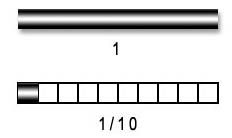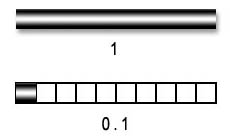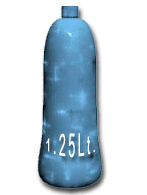Teachers often say that the decimal point shows where the whole number portion ends and where the fraction part begins. However, we need to appreciate that the two parts go together to make the one number. To demonstrate this, we can take the example of a 1.25 Litre bottle of milk. Although there are two parts to the number 1.25, it represents one quantity of milk.

Terminology

We are using the name "decimal" or "decimal number" to refer to a number written in the notation that uses a decimal point. These are sometimes called "decimal fractions", which indicates that they serve a similar function to common fractions.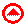Recording and naming decimals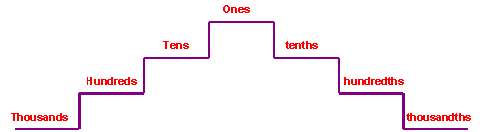This model shows the symmetry around the 'ones' place value column. It does not show the relative size of the columns.

The names of the decimal place value columns reflect those of whole numbers. The decimal point is important in recording and naming decimals because it shows where the ones column is, as it is placed directly after it.

When reading decimals, we can name them according to their place value, and should do so in our teaching role, but generally we read them digit by digit.

## Symbolic Recording

0.5

5 tenths

Zero point five OR Point five

2.89

2 and 8 tenths and 9 hundredths

Two point eight nine

5.253

5 and 2 tenths and 5 hundredths and 3 thousandths

Five point two five threeBasic equivalences

The number 2.89 can be read as 2 units and 8 tenths and 9 hundredths. However, it can also be read as 2 and 89 hundredths. This is because 8 tenths is equal to 80 hundredths. The base 10 properties make these equivalences easy.

Another example:

5.253 = 5 and 2 tenths and 5 hundredths and 3 thousandths = 5 + 0.2 + 0.05 + 0.003

5.253 = 5 and 253 thousandths = 5 + 0.253

5.253 = 5 and 2 tenths and 53 thousandths = 5 + 0.2 + 0.053

5.253 = 5 and 25 hundredths and 3 thousandths = 5 + 0.25 + 0.003

5.253 = 5253 thousandths

The number expander (see below) illustrates these relationships in a memorable way.Models of decimals

Decimals can be represented using various models that allow us to see the relationship between the whole unit and its fractional components. Models also allow us to visualise and gain a greater understanding of place value.

Linear Arithmetic Blocks

Linear Arithmetic Blocks (LAB) provide a physical model of decimal fractions. Each component is one tenth of the length of the previous component. Because the blocks can be arranged in a linear fashion, the size of different decimals can be easily seen and compared.

 One Tenth Hundredth Thousandth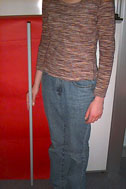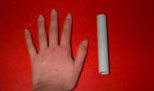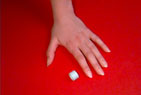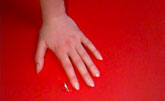Below is shown how the number 0.26 can be represented with LAB.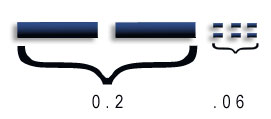Number Expanders

Using a number expander, children can see how numbers can be named differently according to the number of ones, tenths, hundredths and so on.

Below we can see that 3.145 can be represented in many different ways.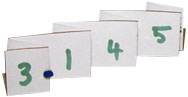3.145 is equal to,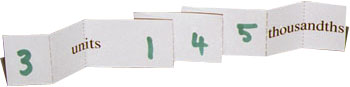3 ones and 145 thousandths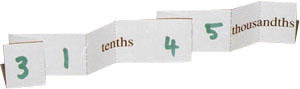31 tenths and 45 thousandths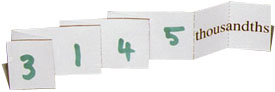3145 thousandths

Measurement Models

Common units of measurement provide good models of decimals. Different measuring devices for length, such as rulers and tape measures, divide a metre into tenths (decimetres) hundredths (centimetres) and thousandths (millimetres). The man in the picture is 174 centimetres tall. This is 1.74 metres or 1740 millimetres.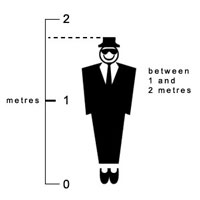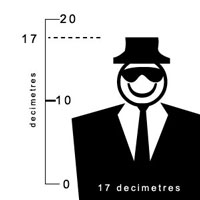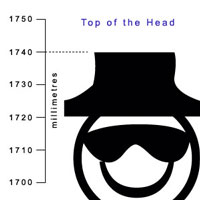Decimals are especially useful in dealing with the continuous quantities often encountered in measurement. Both the metric system and decimals divide units into tenths, hundredths and thousandths, making decimals completely compatible with the metric system. The following example illustrates this.

Example 1:

Joe and Mary are comparing the distances of their jumps in the long jump pit. Both jumps were clearly over three metres, but to see which one is further, they need to look at the portion of the fourth metre of each jump. On a tape measure a metre is divided into one hundred parts (called centimetres), which are small enough to give an accurate measurement of each jump. Joe measured his jump and found that it was 3 metres and 27 hundredths of a metre. Mary's jump measured 3 metres and 40 hundredths of a metre, so hers was the longest.

They recorded these distances as follows:

 Joe 3.27 m 327 cm Mary 3.40 m 340 cm

Meaning and models quiz

Write these as decimals?

 1 15 tenths 2 63 thousandths 3 8 ten thousandths 4 5 ones + 16 tenths + 3 hundredths 5 7 tenths + 89 hundredths + 21 thousandths

Extra questions:

1. Which digit is in the thousandths column in the number 73.9128?

2. What is the place value of 9 in the number 213.196?

3. Shade 0.15 of the following table.

4. Shade 0.2 of the following table

5. What number is one tenth more than 0.4?

6. What number is two hundredths less than 0.837?

7. A box contains a decimal point and the digits 0 to 9, all on separate cards. I draw three cards from the box. These cards contain the decimal point, a four and a zero. What is the smallest number I can make using these three cards if I lie the cards side by side?

8. If a chocolate is cut into ten equal sized pieces and you eat three of these pieces, what decimal fraction of the chocolate will you have eaten?

9. A jigsaw puzzle had 1000 pieces. If three pieces of the jigsaw puzzle get lost what decimal fraction of the jigsaw puzzle is lost?

10. How many hundredths in 1.01?

11. Which is larger, 0.7 or 0.0007?

12. Write 0.9 as a fraction.

13. Write 0.003 as a fraction.

14. Find the missing numbers in the following pattern 0.1, 0.2, ______, 0.4

15. Find the missing numbers in the following pattern 0.27, 0.3, _____, _______

16. The distance from Caitlin’s house to school is 0.4 km and the distance from Jason’s house to school is 0.37 km. Who lives further from the school?

17. Joan is 1.53 m tall and Greg is 1.5 m tall. Who is taller?

18. Which is smaller 3 litres and 56 hundredths of a litre or 3 litres and 4 tenths of a litre?

19. There are 10 000 people living in a village. If 5 of these people are over 70 years of age, what decimal fraction of the people in this village are over 70?

20. Twelve cats out of 100 are ginger.

(a) Express the number of ginger cats in hundredths

(b) Express the number of ginger cats in tenths and hundredths

(c) Write the number of ginger cats as a decimal.